# SchoolChalao

+91-8058868746
• Helpline: +91-8058868746
• Mail us: help@schoolchalao.com
• LOGIN | REGISTER

# Set theory symbols

 Symbol Symbol Name Meaning / definition Example { } set a collection of elements A = {3,7,9,14}, B = {9,14,28} A ∩ B intersection objects that belong to set A and set B A ∩ B = {9,14} A ∪ B union objects that belong to set A or set B A ∪ B = {3,7,9,14,28} A ⊆ B subset A is a subset of B. set A is included in set B. {9,14,28} ⊆ {9,14,28} A ⊂ B proper subset / strict subset A is a subset of B, but A is not equal to B. {9,14} ⊂ {9,14,28} A ⊄ B not subset set A is not a subset of set B {9,66} ⊄ {9,14,28} A ⊇ B superset A is a superset of B. set A includes set B {9,14,28} ⊇ {9,14,28} A ⊃ B proper superset / strict superset A is a superset of B, but B is not equal to A. {9,14,28} ⊃ {9,14} A ⊅ B not superset set A is not a superset of set B {9,14,28} ⊅ {9,66} 2A power set all subsets of A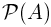power set all subsets of A A = B equality both sets have the same members A={3,9,14}, B={3,9,14}, A=B Ac complement all the objects that do not belong to set A A \ B relative complement objects that belong to A and not to B A = {3,9,14}, B = {1,2,3}, A-B = {9,14} A - B relative complement objects that belong to A and not to B A = {3,9,14}, B = {1,2,3}, A-B = {9,14} A ∆ B symmetric difference objects that belong to A or B but not to their intersection A = {3,9,14}, B = {1,2,3}, A ∆ B = {1,2,9,14} A ⊖ B symmetric difference objects that belong to A or B but not to their intersection A = {3,9,14}, B = {1,2,3}, A ⊖ B = {1,2,9,14} a∈A element of set membership A={3,9,14}, 3 ∈ A x∉A not element of no set membership A={3,9,14}, 1 ∉ A (a,b) ordered pair collection of 2 elements A×B cartesian product set of all ordered pairs from A and B |A| cardinality the number of elements of set A A={3,9,14}, |A|=3 #A cardinality the number of elements of set A A={3,9,14}, #A=3aleph-null infinite cardinality of natural numbers setaleph-one cardinality of countable ordinal numbers set Ø empty set Ø = { } C = {Ø}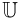universal set set of all possible values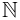0 natural numbers / whole numbers set (with zero)0 = {0,1,2,3,4,...} 0 ∈01 natural numbers / whole numbers set (without zero)1 = {1,2,3,4,5,...} 6 ∈1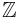integer numbers set= {...-3,-2,-1,0,1,2,3,...} -6 ∈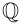rational numbers set= {x | x=a/b, a,b∈} 2/6 ∈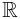real numbers set= {x | -∞ < x <∞} 6.343434∈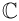complex numbers set= {z | z=a+bi, -∞

Very Useful (0)

Useful (0)

Not Useful (0)